Modulation and Demodulation Using Complex Envelope

This example simulates the different steps of a basic communication process. Communication systems work by modulating chunks of information into a higher carrier frequency, transmitting the modulated signals through a noisy physical channel, receiving the noisy waveforms, and demodulating the received signals to reconstruct the initial information.

All the information carried in a real-valued signal $\mathit{s}\left(\mathit{t}\right)$ can be represented by a corresponding lowpass complex envelope:

$\mathit{s}\left(\mathit{t}\right)=\mathrm{Re}\left\{\mathit{g}\left(\mathit{t}\right){\mathit{e}}^{\mathit{j}2\pi {\mathit{f}}_{\mathrm{c}}\mathit{t}}\right\}=\mathit{i}\left(\mathit{t}\right)\mathrm{cos2}\text{\hspace{0.17em}}\pi \text{\hspace{0.17em}}{\mathit{f}}_{\mathrm{c}}\mathit{t}-\mathit{q}\left(\mathit{t}\right)\mathrm{sin2}\text{\hspace{0.17em}}\pi \text{\hspace{0.17em}}{\mathit{f}}_{\mathrm{c}}\mathit{t}$.

In this equation:

• ${\mathit{f}}_{\mathrm{c}}$ is the carrier frequency.

• $Re$ represents the real part of a complex-valued quantity.

• $\mathit{g}\left(\mathit{t}\right)=\mathit{i}\left(\mathit{t}\right)+\mathit{jq}\left(\mathit{t}\right)$is the complex envelope of $\mathit{s}\left(\mathit{t}\right)$.

• $\mathit{i}\left(\mathit{t}\right)$ is the inphase component of the complex envelope.

• $\mathit{q}\left(\mathit{t}\right)$ is the quadrature component of the complex envelope.

The complex envelope is modulated to the carrier frequency and sent through the channel. At the receiver, the noisy waveform is demodulated using the carrier frequency.

The phase variation due to the carrier frequency is predictable and thus does not convey any information. The complex envelope does not include the phase variation and can be sampled at a lower rate.

Generate a signal whose complex envelope consists of a sinusoid and a chirp. The inphase component is a sinusoid with a frequency of 19 Hz. The quadrature component is a quadratic chirp whose frequency ranges from 61 Hz to 603 Hz. The signal is sampled at 2 kHz for 1 second.

fs = 2e3;
t = (0:1/fs:1-1/fs)';
inph = sin(2*pi*19*t);

Compute the complex envelope and store it as a MATLAB® timetable of sample rate fs.

g = timetable(env,'SampleRate',fs);

Open Signal Analyzer and drag the complex envelope from the Workspace browser to the Signal table. The display shows the inphase and quadrature components of the envelope as lines of the same hue and saturation, but different luminosity. The first line color represents the inphase component and the second line color represents the quadrature component. Click Spectrum ▼ on the Display tab and select Spectrum. The app displays a set of axes with the signal spectrum. The complex envelope has a two-sided spectrum, displayed as a line of the same color of the inphase component of the complex envelope.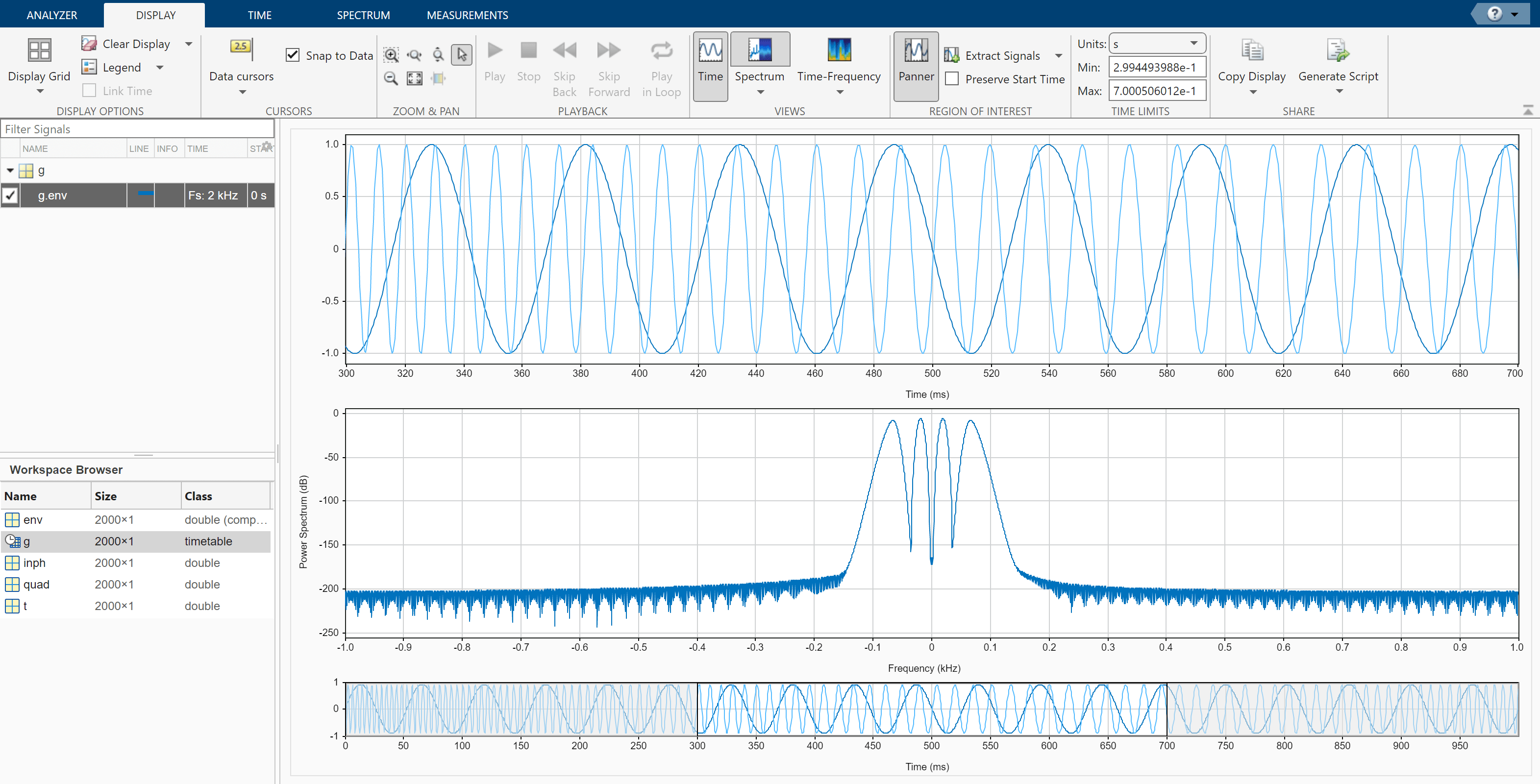On the Display tab, click Panner to activate the panner. Use the panner to create a zoom window between 300 ms and 720 ms. Drag the zoom window so that it is centered at 0 Hz. The spectrum has an impulse at 0.19 kHz and a wider tapering profile at higher frequencies. The negative-frequency region of the spectrum is a mirror image of the positive-frequency region.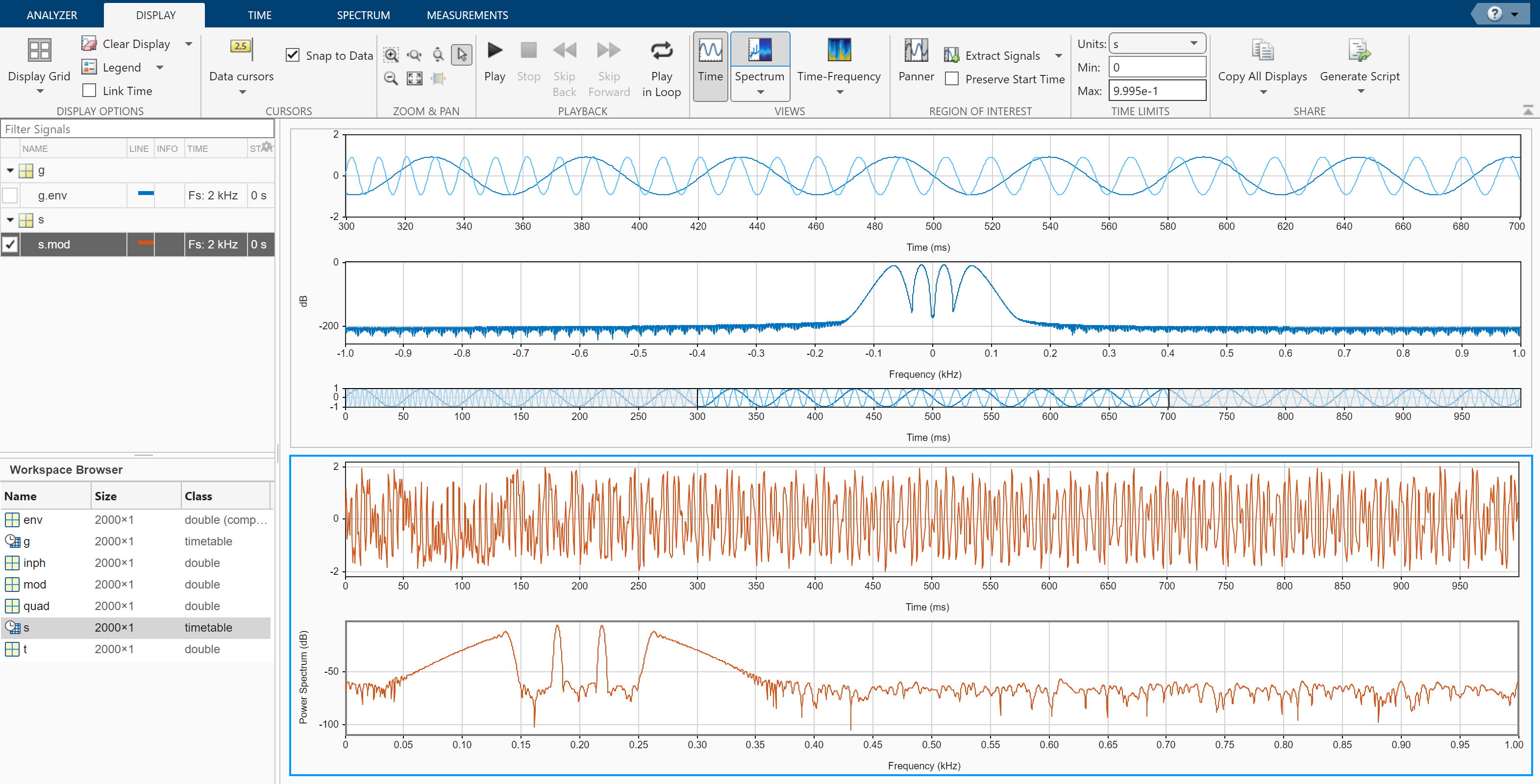Modulate the signal using a carrier frequency of 200 Hz. Multiply by $\sqrt{2}$ so that the power of the modulated signal equals the power of the original signal. Add white Gaussian noise such that the signal-to-noise ratio is 40 dB.

fc = 200;
mod = sqrt(2)*real(env.*exp(2j*pi*fc*t));

SNR = 40;
mod = mod + randn(size(mod))*std(mod)/db2mag(SNR);
s = timetable(mod,'SampleRate',fs);

Click Display Grid ▼ to add a second display. Drag the modulated signal to the Signal table and enter the time information. The modulation has moved the spectrum to positive frequencies centered on the carrier frequency.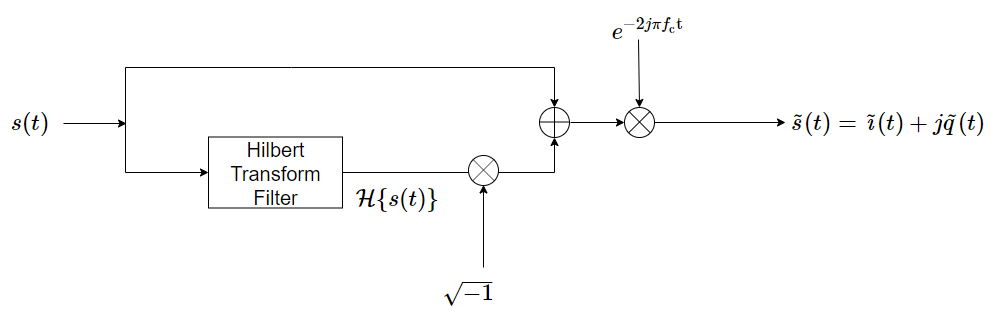Calculate the analytic signal and demodulate the signal by multiplying the analytic signal with a complex-valued negative exponential of frequency 200 Hz.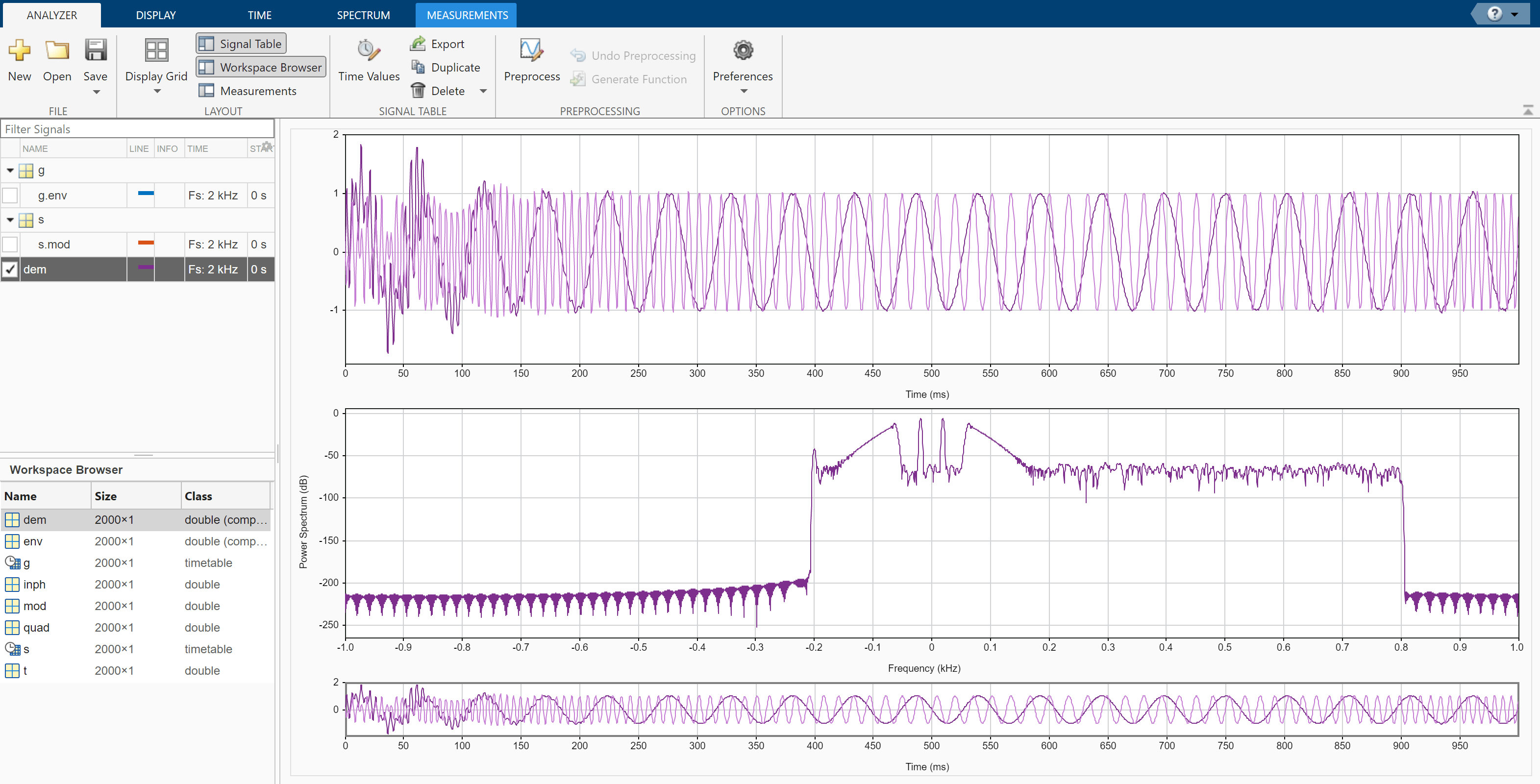dem = hilbert(mod).*exp(-2j*pi*fc*t)/sqrt(2);

Click Display Grid ▼ to create a three-by-one grid of displays. Drag the demodulated signal to the Signal table. Add time information to the complex envelope by clicking Time Values in the Analyzer tab. The two-sided spectrum shows the recovered inphase and quadrature components of the baseband signal.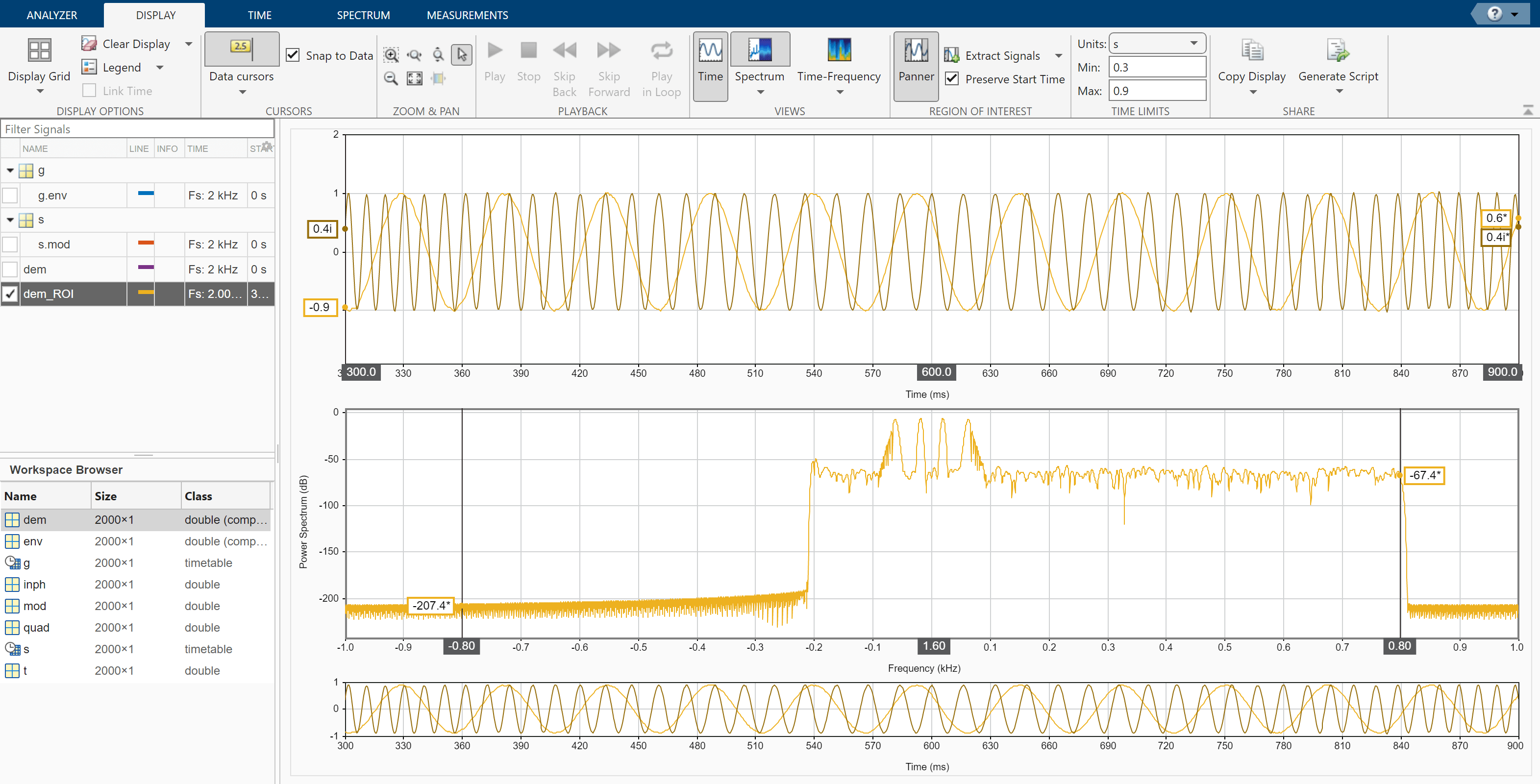Click Display Grid ▼ to create a one-by-one grid of displays and plot the demodulated signal. Click Data Cursors ▼ and select Two. Place the time-domain cursors at 300 ms and 900 ms, so they enclose the spectral peaks. Click Extract Signals ▼ and select Between Time Cursors. Check the Preserve Start Time box. Clear the display and plot the extracted signal. The app extracts both inphase and quadrature components of the demodulated signal in the region of interest. Select the extracted signal by clicking its Name column in the Signal table. On the Analyzer tab, click Export and save the signal to a MAT-file called dem_ROI.mat.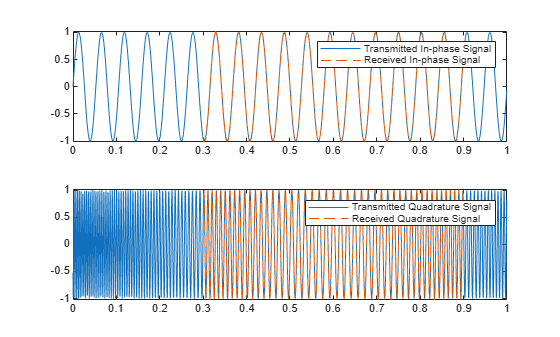Load the dem_ROI file to the workspace. Compute the demodulated inphase and quadrature components by taking the real and imaginary parts of the extracted signal. Store the time information of the extracted signal in a time variable t_dem.

inph_dem = real(dem_ROI);
t_dem = 0.3+(0:length(dem_ROI)-1)/fs;

Compare the transmitted waveforms and the extracted regions of interest. Also compare their spectra.

subplot(2,1,1)
plot(t,inph,t_dem,inph_dem,'--')

subplot(2,1,2)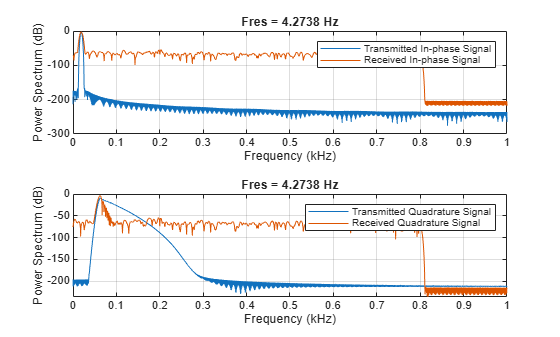figure
subplot(2,1,1)
pspectrum(inph,fs)
hold on
pspectrum(inph_dem,fs)
hold off

subplot(2,1,2)
hold on
hold off